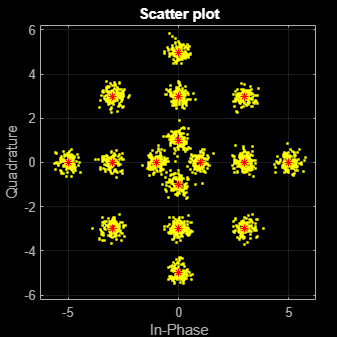# General QAM Modulation in AWGN Channel

Transmit and receive data using a nonrectangular 16-ary constellation in the presence of Gaussian noise. Show the scatter plot of the noisy constellation and estimate the symbol error rate (SER) for two different signal-to-noise ratios.

Create a 16-QAM constellation based on the V.29 standard for telephone-line modems.

```c = [-5 -5i 5 5i -3 -3-3i -3i 3-3i 3 3+3i 3i -3+3i -1 -1i 1 1i]; M = length(c);```

Generate random symbols.

`data = randi([0 M-1],2000,1);`

Modulate the data by using the `genqammod` function. General QAM modulation is necessary because the custom constellation is not rectangular.

`modData = genqammod(data,c);`

Pass the signal through an AWGN channel having a 20 dB signal-to-noise ratio (SNR).

`rxSig = awgn(modData,20,'measured');`

Display a scatter plot of the received signal and the reference constellation, `c`.

```h = scatterplot(rxSig); hold on scatterplot(c,[],[],'r*',h) grid hold off```Demodulate the received signal by using the `genqamdemod` function. Determine the number of symbol errors and the symbol error ratio.

```demodData = genqamdemod(rxSig,c); [numErrors,ser] = symerr(data,demodData)```
```numErrors = 1 ```
```ser = 5.0000e-04 ```

Repeat the transmission and demodulation process with an AWGN channel having a 10 dB SNR. Determine the symbol error rate for the reduced SNR. As expected, the performance degrades when the SNR is decreased.

```rxSig = awgn(modData,10,'measured'); demodData = genqamdemod(rxSig,c); [numErrors,ser] = symerr(data,demodData)```
```numErrors = 462 ```
```ser = 0.2310 ```